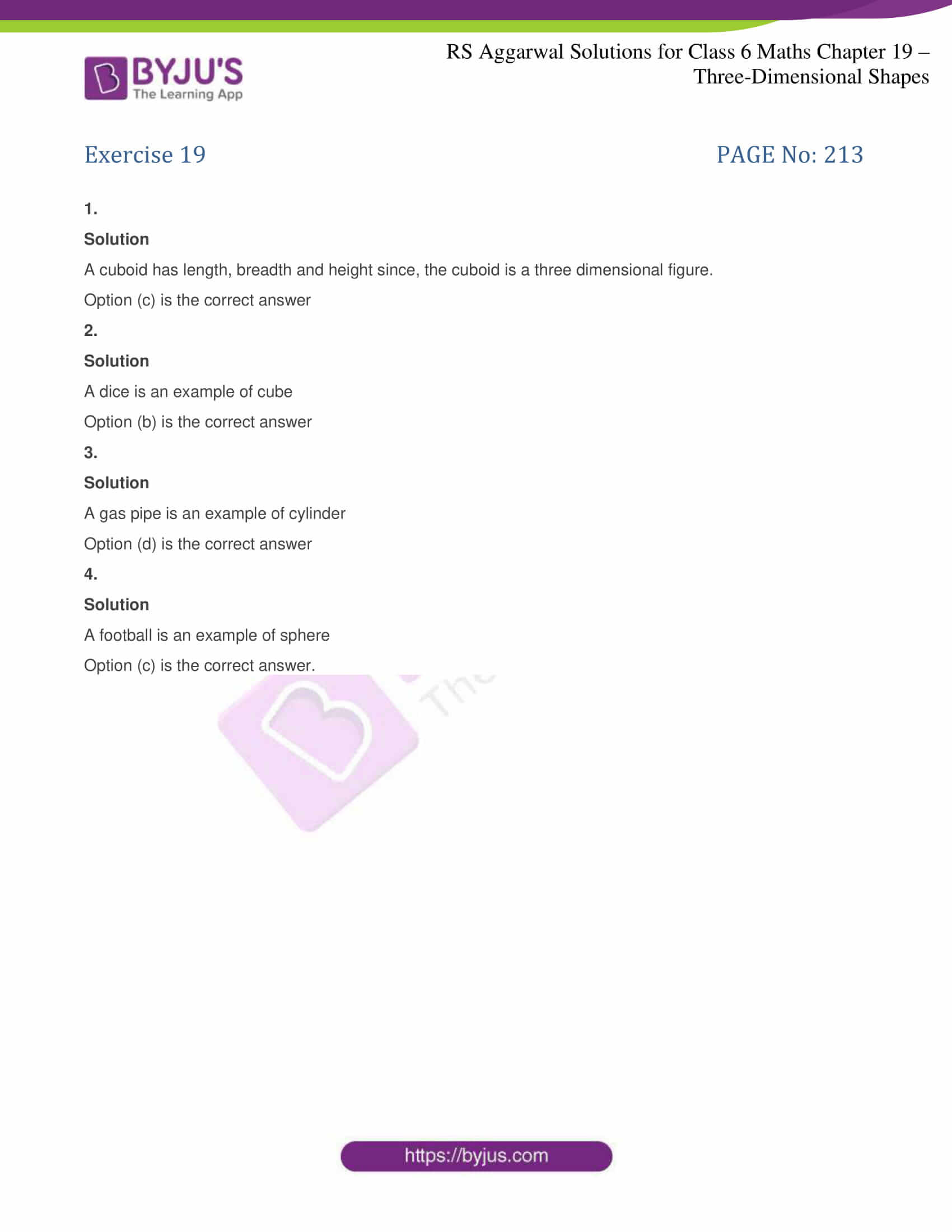# RS Aggarwal Solutions for Class 6 Maths Chapter 19 Three-Dimensional Shapes

RS Aggarwal Solutions for Class 6 Maths Chapter 19 Three-Dimensional Shapes are provided here for students. By diligently practicing RS Aggarwal textbook questions, students score high marks, and thereby excel in the examinations. Going through RS Aggarwal Solutions students understand the chapter effectively without any doubts.

Simple PDF format of RS Aggarwal textbook is available which has been solved by experts at BYJU’S to help all the students. Download PDF of Class 6 Chapter 19 in their respective links.

## Download PDF of RS Aggarwal Solutions for Class 6 Maths Chapter 19### RS Aggarwal Solutions for Class 6 Maths Chapter 19 – Three-Dimensional Shapes

Chapter 19 – Three-Dimensional Shapes consists of 1 Exercise. Each question of RS Aggarwal textbook has been solved perfectly. Let’s have a look at the topics which are given here in this chapter.

• Cuboid
• Cube
• Cylinder
• Sphere
• Cone
• Triangular Prism
• Pyramid

A solid figure or shape that has three dimensions, length, width and height is known as Three-Dimensional Shape. Faces, edges and vertices are the attributes of Three-Dimensional Shapes. Cube, cone, prism, cylinder, square, pyramid, sphere are examples of Three-Dimensional Shapes.